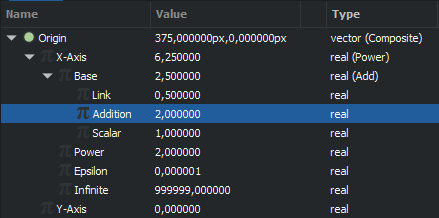# Mathematical equation typing

Is there a way we can type mathematical equations on synfig? like the formula of (a+b)^2 etc…,

No, not directly.
You will need to adapt your formulas into Synfig’s converters: Convert - Synfig Animation Studio

For example, for your formula you need ‘Power’ and ‘Add’ converters, so conversion will look like this (substitute “Origin” with parameter you need):1 Like

Wow. Looks pretty complex. Will try it out though!
Thanks!!